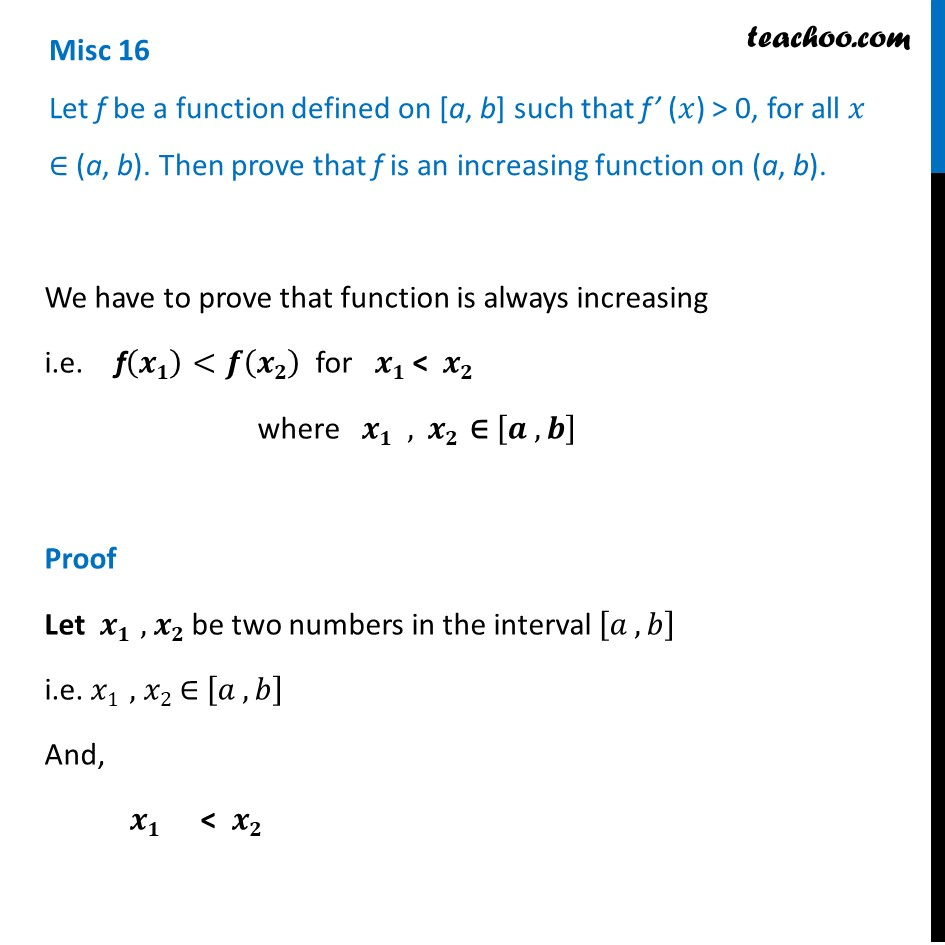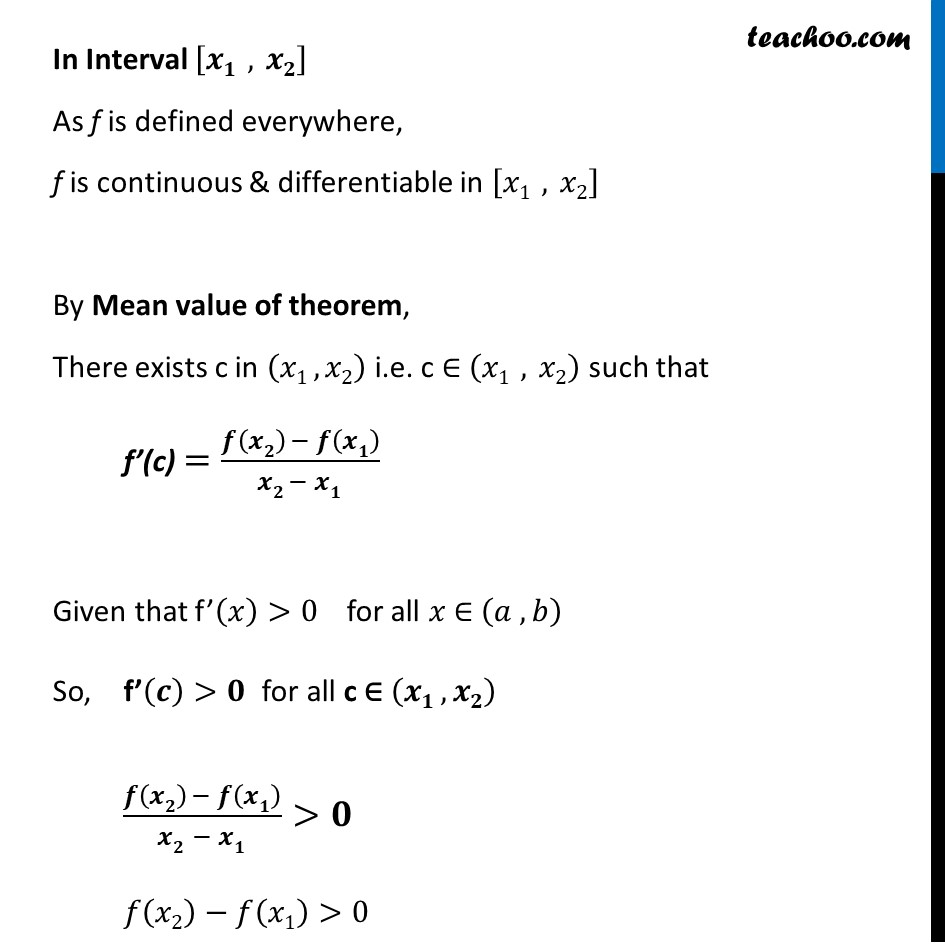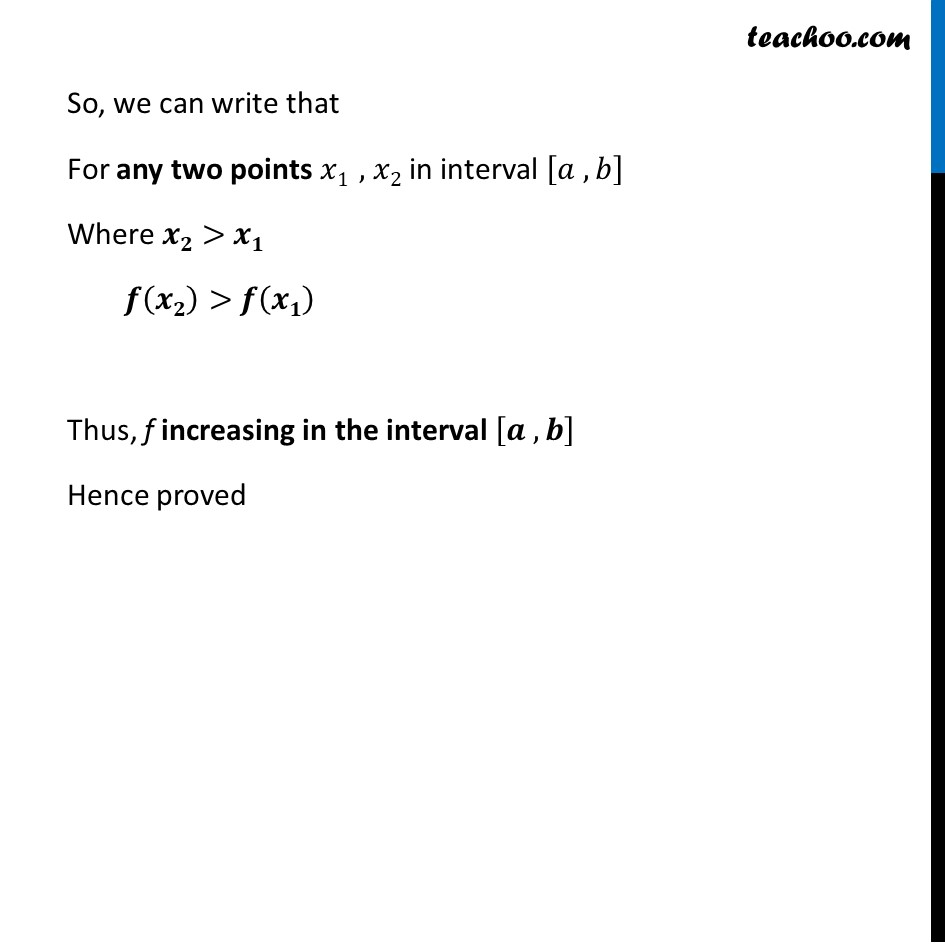Miscellaneous

Chapter 6 Class 12 Application of Derivatives (Term 1)
Serial order wise### Transcript

Misc 16 Let f be a function defined on [a, b] such that f’ (𝑥) > 0, for all 𝑥 ∈ (a, b). Then prove that f is an increasing function on (a, b).We have to prove that function is always increasing i.e. f(𝒙𝟏)<𝒇(𝒙𝟐) for 𝒙𝟏 < 𝒙𝟐 where 𝒙𝟏 , 𝒙𝟐 ∈ [𝒂 , 𝒃] Proof Let 𝒙𝟏 , 𝒙𝟐 be two numbers in the interval [𝑎 , 𝑏] i.e. 𝑥1 , 𝑥2 ∈ [𝑎 , 𝑏] And, 𝒙𝟏 < 𝒙𝟐 In Interval [𝒙𝟏 ," " 𝒙𝟐] As f is defined everywhere, f is continuous & differentiable in [𝑥1 ," " 𝑥2] By Mean value of theorem, There exists c in (𝑥1 ,𝑥2) i.e. c ∈ (𝑥1 ," " 𝑥2) such that f’(c) =(𝒇(𝒙𝟐) − 𝒇(𝒙𝟏))/(𝒙𝟐 − 𝒙𝟏 ) Given that f’(𝑥)>0 for all 𝑥 ∈ (𝑎 , 𝑏) So, f’(𝒄)>𝟎 for all c ∈ (𝒙𝟏 ,𝒙𝟐) (𝒇(𝒙𝟐) − 𝒇(𝒙𝟏))/(𝒙𝟐 − 𝒙𝟏 )>𝟎 𝑓(𝑥2)−𝑓(𝑥1)>0 So, we can write that For any two points 𝑥1 , 𝑥2 in interval [𝑎 , 𝑏] Where 𝒙𝟐> 𝒙𝟏 𝒇(𝒙𝟐)> 𝒇(𝒙𝟏) Thus, f increasing in the interval [𝒂 , 𝒃] Hence proved